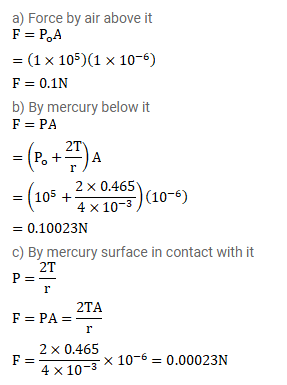# Consider a small surface area of`
Question:

Consider a small surface area of $1 \mathrm{~mm}^{2}$ at the top of a mercury drop of radius $4.0 \mathrm{~mm}$. Find the force exerted on this area

(a) by the air above it

(b) by the mercury below it and

(c) By the mercury surface in contact with it.

Atmospheric pressure $=1.0 \times 10^{6} \mathrm{~Pa}$ and surface tension of mercury $=0.465 \mathrm{~N} / \mathrm{m}$. Neglect the effect of gravity. Assume all numbers to be exact.

Solution: